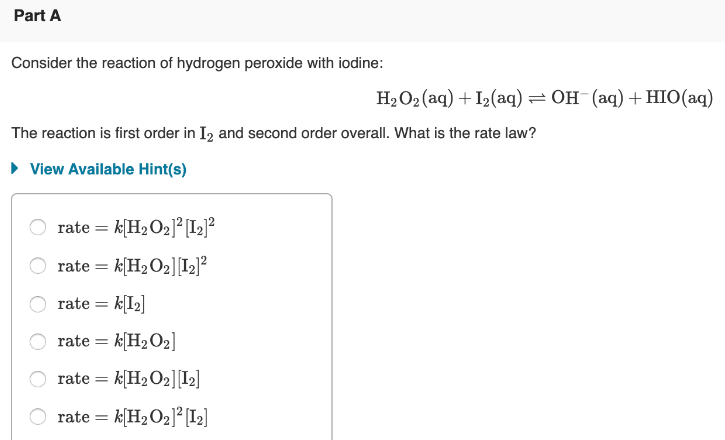# Consider the reaction of hydrogen peroxide with iodine: H2O2 (aq) + I2(aq) ⇌ OH^- (aq) + HIO (aq) The reaction is first order in I2 and second order overall. What is the rate law? a) rate = k[H2O2]^2[I2]^2 b) rate = k[H2O2][I2]^2 c) rate = k[I2] d) rate = k[H2O2] e) rate = k[H2O2][I2] f) rate = k[H2O2]^2[I2]